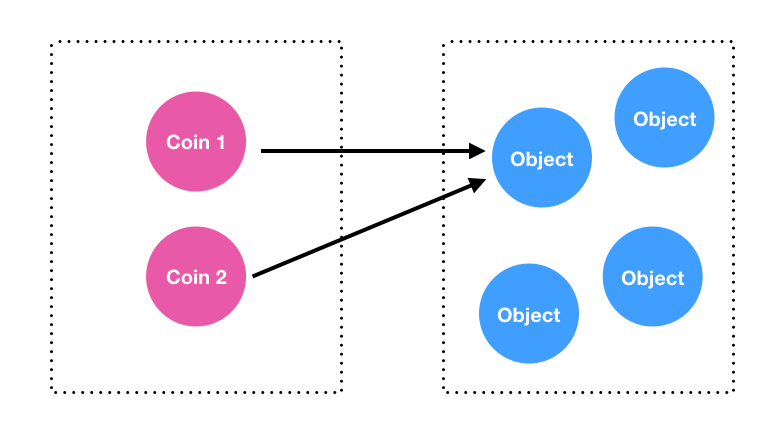#2018 iT 邦幫忙鐵人賽DAY 5
16
Modern Web

## 基本型別

``````var a = 10;
var b = 10;

// 在 JavaScript 判斷是否「相等」用 " === "
// 後續提到運算子會詳細介紹。

console.log( a === b );      // true
``````

``````var a = 'Kuro';
var b = 'Kuro';
var c = 'Jack';

console.log( a === b );      // true
console.log( a === c );      // false

``````

## 物件型別

``````var obj1 = { value: 10 };
var obj2 = { value: 10 };
``````

.
.
.

``````var a = 10;
var b = 10;

console.log( a === b );      // true
``````

``````// 兩個 coin 的價值都是 10，但 coin1 與 coin2 卻不是同一個實體。
var coin1 = { value: 10 };
var coin2 = { value: 10 };

console.log( coin1 === coin2 );      // false

// 我在 coin1 畫了一個 X
coin1.cross = true;

// coin2.cross 當然不可能會有東西
console.log( coin2.cross );          // undefined
``````

## 變數的更新與傳遞

### 基本型別的更新與傳遞

``````var a = 10;
var b = a;

console.log( a );   // 10
console.log( b );   // 10
``````

``````a = 100;

// 變數 b 依然是 10，而變數 a 變成了 100
console.log( a );   // 100
console.log( b );   // 10
``````

### 物件型別的更新與傳遞

``````var coin1 = { value: 10 };
var coin2 = coin1;

console.log( coin1.value );       // 10
console.log( coin2.value );       // 10
``````

``````coin1.value = 100;

console.log( coin1.value );       // 100
console.log( coin2.value );       // 100
``````

`coin1.value` 的內容被更新了之後，連帶著 `coin2.value` 卻也跟著更新了。

``````console.log( coin1 === coin2 );    // true
````````````var coin1 = { value: 10 };
````````````var coin2 = coin1;
``````

``````coin1.value = 100;

console.log( coin1.value );       // 100
console.log( coin2.value );       // 100
``````

## 「傳值」或「傳址」？

``````var coin1 = { value: 10 };

function changeValue(obj) {
obj = { value: 123 };
}

changeValue(coin1);
console.log(coin1);   // ？
``````

「傳值」或「傳址」對大多數的開發者來說應該都不陌生，那麼「pass by sharing」又是什麼呢？

## Pass by sharing

「Pass by sharing」的特點在於，當 `function` 的參數，如 `function changeValue(obj){ ... }` 中的 `obj` 被重新賦值的時候，外部變數的內容是不會被影響的。

``````var coin1 = { value: 10 };

function changeValue(obj) {
obj = { value: 123 };
}

changeValue(coin1);
console.log(coin1);   // 此時 coin1 仍是 { value: 10 }
``````

``````var coin1 = { value: 10 };

function changeValue(obj) {
// 僅更新 obj.value，並未重新賦值
obj.value = 123;
}

changeValue(coin1);
console.log(coin1);   // 此時 coin1 則會變成 { value: 123 }
``````

## Pass by value、 Pass by reference 、Pass by sharing

Regardless of usage concept of reference in this case, this strategy should not be confused with the “call by reference” discussed above. The value of the argument is not a direct alias, but the copy of the address.

``````var a = 10;
var b = a;

a = 100;

console.log(a);     // 100
console.log(b);     // 10
``````

### 4 則留言

00

「傳值」或「傳址」對大多數的開發者來說應該都不陌生，那麼「psaa by sharing」又是什麼呢？

psaa → pass

Kuro Hsu iT邦新手 3 級 ‧ 2017-12-11 10:00:21 檢舉

0

0

It can be either “call by value”, with specifying that the special case of call by value is meant — when the value is the address copy. From this position it is possible to say that everything in ECMAScript is passed by value.
Or, “call by sharing”, which makes this distinction from “by reference”, and “by value”. In this case it is possible to separate passing types: primitive values are passed by value and objects — by sharing.

(1) “passed by value”（特殊情況時，value 是指 address copy）
(2) primitive 是 “passed by value” ＋ objects 是 “passed by sharing”